Latest Banking jobs   »

# Quantitative Aptitude Quiz For IDBI AM/ Bank of India PO 2023-10th March

Q1. Ratio of difference between area of a rectangle obtained in two cases, first when length of a rectangle is decreased by 4 cm and second when breadth of the original rectangle is increased by 4 cm to the area of rectangle is 4 : 9. Find ratio of numerical value perimeter to area of rectangle.
(a) 2 : 7
(b) 2 : 5
(c) 2 : 11
(d) 2 : 9
(e) None of these
Q2. 24 men can complete a work in 20 days. 36 women can do the same work in 40 days. 54 children can do that work in 40 days. 18 women and 18 children together do that work for 32 days and ‘X’ number of men complete the remaining work in four days, then find (X + 14) women & (X -13) child can do the same work in how many days?
(a) 16 days
(b) 24 days
(c) 20 days
(d) 30 days
(e) 36 days
Q3. Shatabdhi express travelling at 168 kmph and overtook Rajdhani express, traveling in the same direction in 20 seconds. If Rajdhani express had been traveling at twice its speed, then Shatabdhi express would have taken 45 seconds to overtake it. Find the length of Shatabdi express, given that its length is twice of the length of Rajdhani express?
(a) 180 m
(b) 720 m
(c) 360 m
(d) 400 m
(e) 200 m
Q4. A solid sphere iron ball having radius of 12 cm melted and re- casted into a hollow cylindrical vessel of uniform thickness. If external radius of the base of cylindrical vessel is 10 cm and its height is 64 cm, then find the uniform thickness of the cylindrical vessel?
(a) 2 cm
(b) 1 cm
(c) 2.5 cm
(d) 2.25 cm
(e) None of these
Q5. Anurag and Yuvraj entered into partnership with Rs. 1400 and Rs. 1200 respectively. After three months, Anurag withdrew two-sevenths of his initial investment but after another three months Anurag puts back three-fifth of what he had withdrawn. The total profit at the end of the year is Rs. 14520. Find profit of Anurag?
(a) 7200 Rs.
(b) 7320 Rs.
(c) 14640 Rs.
(d) 7620 Rs.
(e) 7820 Rs.

Q6. From container P containing 108 liters of mixture of milk and water, water is 12.5% of milk in container. 36 liters of the mixture is taken out and poured into container Q in which ratio of milk to water is 3 : 1. If difference between total milk and total water in container Q is 60 liters, then find difference between the quantity of initial mixture in container Q and final mixture in P?
(a) 6 liters
(b) 4 liters
(c) 8 liters
(d) 10 liters
(e) 12 liters
Q7. A man invested an amount at the rate of 20% p.a. on compound interest for two years. After two years he added 75% of the initial amount to the amount obtained and invested this amount at same rate of interest. If man got a total of Rs. 3512 as interest after three years, find the amount received by man after three years, if he invested same amount on simple interest at rate of 15% p.a.?
(a) 5800 Rs.
(b) 5600 Rs.
(c) 5000 Rs.
(d) 5200 Rs.
(e) None of these
Q8. Ankit and Bhavya entered in a business by making investment of Rs. 8000 & Rs. 11000 respectively. After six months Ankit & Bhavya withdrew Rs. 2000 and Rs. 3000 respectively and Anurag joined them with capital of Rs. 4P. If after one year and three months Anurag received Rs. 4500 as profit share out of total profit of Rs. 24500, then find investment of Anurag?
(a) 12000 Rs.
(b) 6000 Rs.
(c) 15000 Rs.
(d) 9600 Rs.
(e) 18000 Rs.
Q9. ‘P’ is a cold drinks seller who marked up price of each cold drink bottle by 75% above C.P. and allow discount of 40% and made a profit of Rs. 15 on each bottle of cold drink. If ‘P’ allows 24% discount on each bottle, then find how much profit seller gets on selling 160 bottles?
(a) 15960 Rs.
(b) 15640 Rs.
(c) 15840 Rs.
(d) 15550 Rs.
(e) None of these
Q10. Ritu invested her total saving in three different FD schemes A, B and C in the ratio of 5 : 4 : 6 on CI for two years at the rate of 10%, 15% and 20% respectively.If interest is calculated annually and interest from scheme B is Rs. 744 more than interest from scheme A then, find difference between interest received from scheme C and scheme B by Ritu?
(a) Rs. 4185
(b) Rs. 4175
(c) Rs. 3840
(d) Rs. 4580
(e) Rs. 3250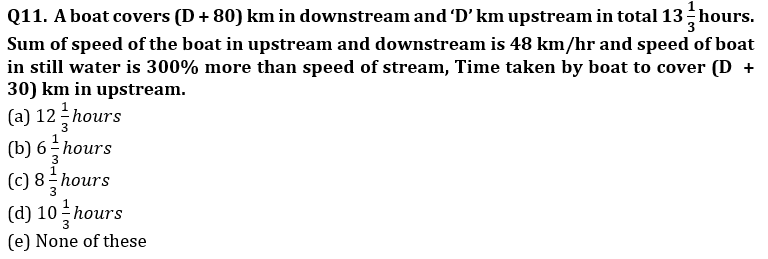Q12. A man ‘X’ invested Rs. 5600 on SI at the rate of 7.5% p.a. for two years and another man ‘Y’ invest Rs. ‘P’ on CI at the rate of 20% p.a. for two years. Ratio of total SI gots by ‘X’ to CI gots by ‘Y’ only for second year is 7 : 9. If X & Y invested his respective amount in a partnership business for 9 months & 12 months respectively and gets total profit of Rs. 5800, then find difference between profit share of X & Y?
(a) 50 Rs.
(b) 200 Rs.
(c) 400 Rs.
(d) 100 Rs.
(e) 600 Rs.

Q13. A vessel contains mixture of Milk & water from which 40% of mixture from vessel is replaced with mango juice. Resulting quantity of water in vessel is 25% more than milk, while resulting quantity of mango juice in vessel is 50% more than milk. If initial quantity of milk in vessel is 10 liters less than water and X% of resulting mixture taken out, then sum of water & mango juice in vessel becomes 33 liters. Find ‘X’?
(a) 50
(b) 60
(c) 40
(d) 35
(e) 25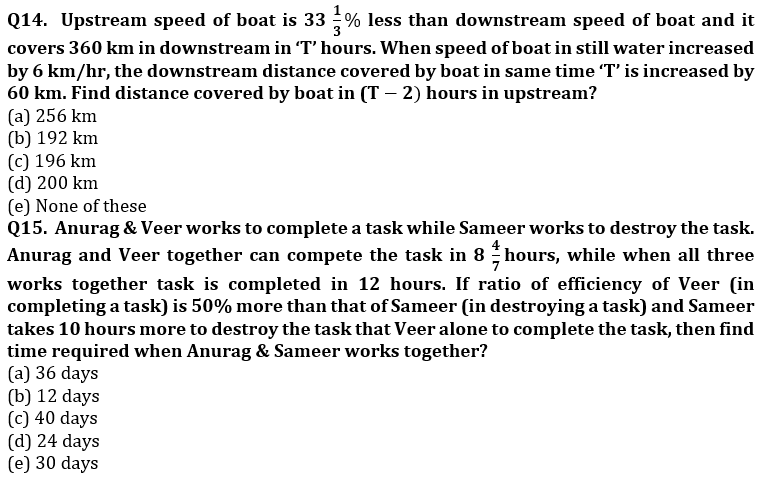Solutions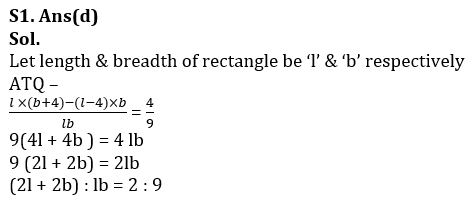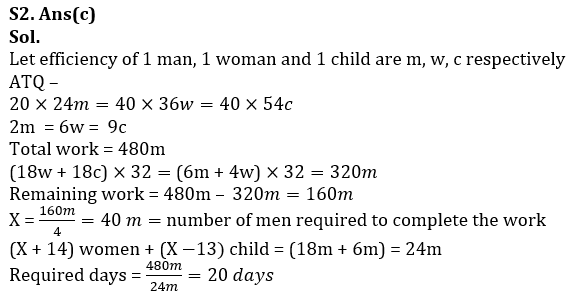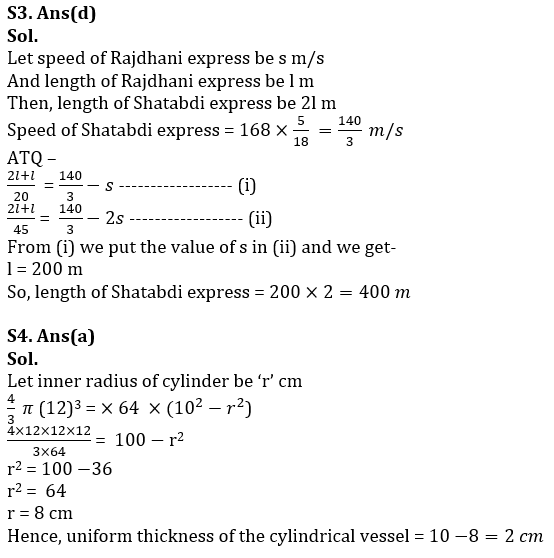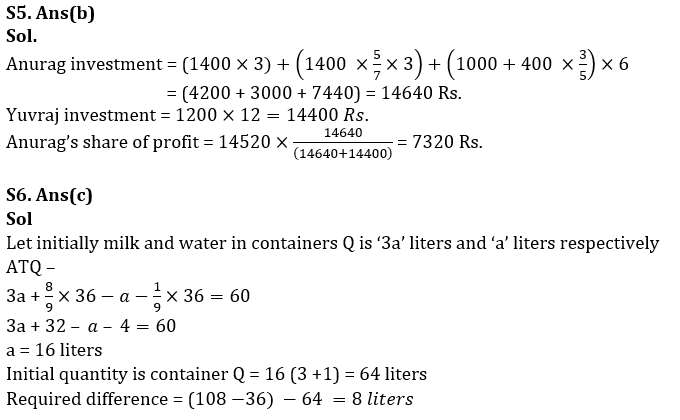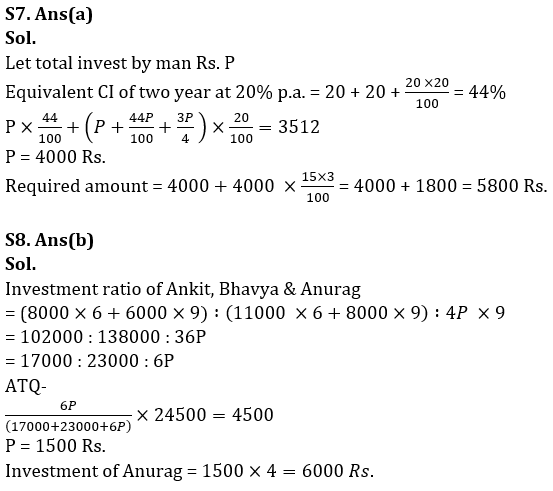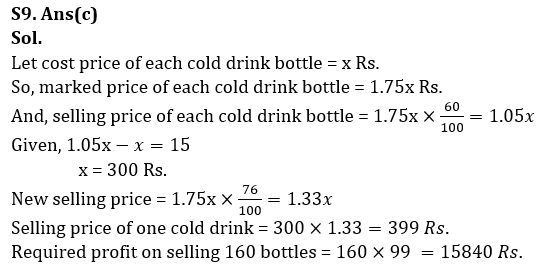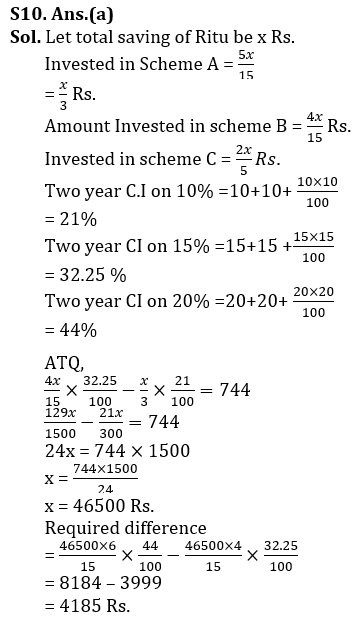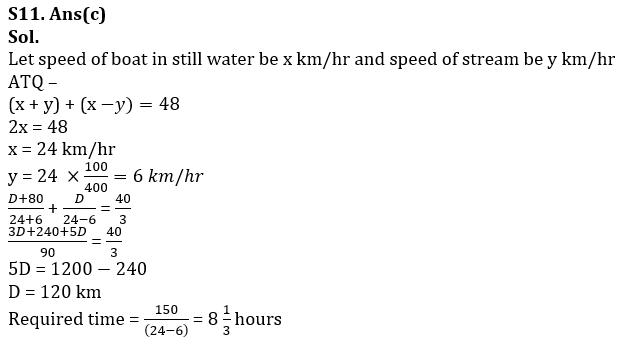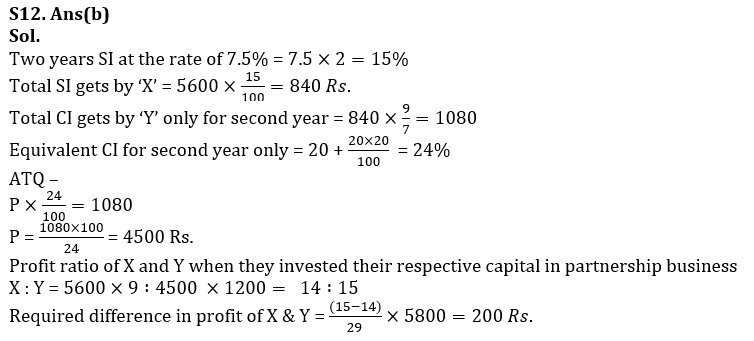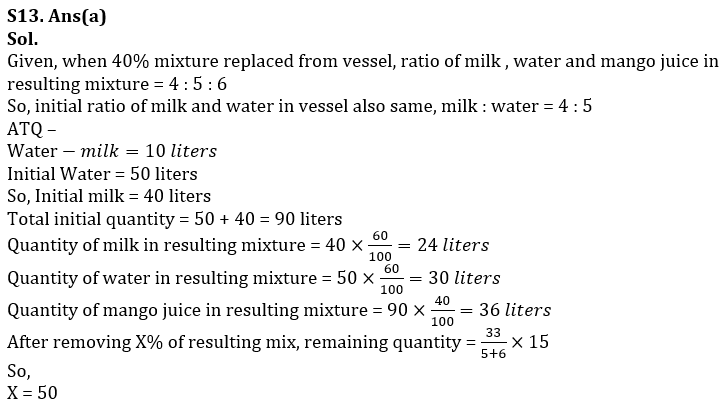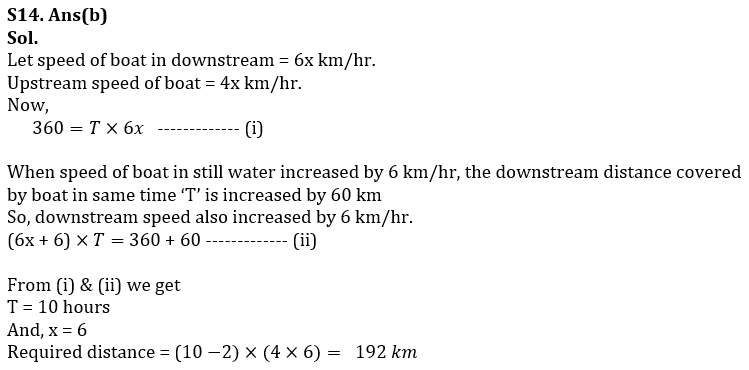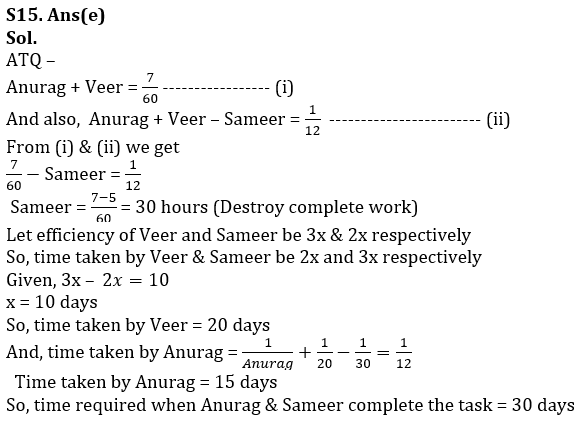## FAQs

### What is the selection process of the IDBI AM?

The selection process of IDBI AM is Online exam and Interview.

#### Congratulations!Union Budget 2023-24: Free PDF